Boolean Algebra & Digital Logic

Boolean algebra was developed by the Englishman George Boole, who published the basic principles in the 1854 treatise An Investigation of the Laws of Thought on Which to Found the Mathematical Theories of Logic and Probabilities.

The applicability to computing machines was discovered by three Americans

Claude Shannon      Symbolic Analysis of Relay and Switching Circuits, 1938.

George Stibitz         An employee of Bell Labs, he developed a binary adder
using mechanical relays in 1937, the model “K 1” adder
because he built it at home on his kitchen table.

John Atanasoff        He was probably the first to use purely electronic relays
(vacuum tubes) to build a binary adder.

Boolean algebra is a two–valued algebra based on the constant values denoted as either

FALSE, TRUE
0, 1

The use of this algebra for computation is based on the fact that binary arithmetic
is based on two values, always called “0” and “1”.

Basic Boolean Operators

Boolean algebra is defined in terms of two constants (defined above), which we
call “0” and “1”.  Other courses will call these values “F” and “T”.

Boolean algebra is defined in terms of three basic operators, to which we shall add
a useful fourth operator.  The three operators are NOT, AND, & OR.

Each of these three basic operators is implemented by a basic electronic device
called a “logic gate”.  We present the gates along with the definition.

NOT       This function takes one input and produces one output.  The gate is shown
below.  The circle at the right end of the triangle is important.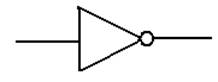Algebraically, this function is denoted f(X) = X’ or f(X) =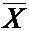The evaluation of the function is simple: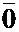= 1 and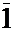= 0.

Basic Boolean Operators (Part 2)

Logic OR

This is a function of two Boolean variables.  We denote the logical OR of two Boolean variables X and Y by “X + Y”.  Some logic books will use “X Ú Y”.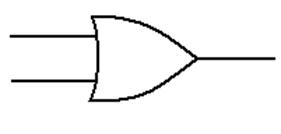The evaluation of the logical OR function is shown by a truth table

 X Y X + Y 0 0 0 0 1 1 1 0 1 1 1 1

Basic Boolean Operators (Part 3)

Logic AND

This is a function of two Boolean variables.  We denote the logical AND of two Boolean variables X and Y by “X · Y”.  Some logic books will use “X Ù Y”.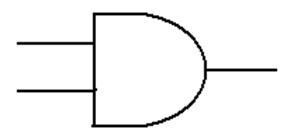The evaluation of the logical AND function is shown by a truth table

 X Y X · Y 0 0 0 0 1 0 1 0 0 1 1 1

Another Boolean Operator

While not a basic Boolean operator, the exclusive OR is very handy.

Logic XOR

This is a function of two Boolean variables.  We denote the logical XOR of two Boolean variables X and Y by “X Å Y”.  Most logic books seem to ignore this function.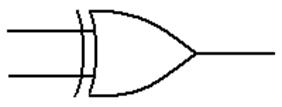The evaluation of the logical XOR function is shown by a truth table

 X Y X Å Y 0 0 0 0 1 1 1 0 1 1 1 0

From this last table, we see immediately that
X
Å 0 = X and X Å 1 =Truth Tables

The fact that any Boolean variable can assume only one of two possibly values can be shown, by induction, to imply the following.

For N > 0, N Boolean variables can take only 2N different combinations of values.

For small values of N, we can use this to specify a function using a truth table with 2N rows, plus a header row to label the variables and the function.

 N 2N 1 2 2 4 3 8 4 16 5 32 6 64

Four–variable truth tables have 17 rows total.  This is just manageable.
Five–variable truth tables have 33 rows total.  This is excessive.
N–variable truth tables, for N > 5, are almost useless.

Sample Truth Table

 A B C F1(A, B, C) 0 0 0 0 0 0 1 1 0 1 0 1 0 1 1 0 1 0 0 1 1 0 1 0 1 1 0 0 1 1 1 1

This truth table for 3 variables has 23 = 8 rows, plus a label row.

This truth table forms a complete definition of the function.  We shall later
give it another name, but can base all our discussions on this table.

Another Sample Truth Table

 A B C F2(A, B, C) 0 0 0 0 0 0 1 0 0 1 0 0 0 1 1 1 1 0 0 0 1 0 1 1 1 1 0 1 1 1 1 1

Two Truth Tables in One

 A B C F1(A, B, C) F2(A, B, C) 0 0 0 0 0 0 0 1 1 0 0 1 0 1 0 0 1 1 0 1 1 0 0 1 0 1 0 1 0 1 1 1 0 0 1 1 1 1 1 1

Truth tables are often used to show pairs of functions, such as these two,
which will later be shown to be related.  This is easier than two complete tables.

Truth tables rarely show more than two functions, just because large truth
tables are “messy” and hard to read.

Labeling Rows in a Truth Table

The row numbers are just labels.  They are not really a part of the truth table, but
aid in our discussions and conversions to Boolean expressions.

The row numbers are the decimal equivalents of the variable values viewed as binary

 Row Number X Y Z F(X, Y, Z) 0 0 0 0 1 1 0 0 1 1 2 0 1 0 0 3 0 1 1 1 4 1 0 0 1 5 1 0 1 0 6 1 1 0 1 7 1 1 1 1

numbers.  The first row is always “row 0”.

0 = 0·4 + 0·2 + 0·1

1 = 0·4 + 0·2 + 1·1

2 = 0·4 + 1·2 + 0·1

3 = 0·4 + 1·2 + 1·1

4 = 1·4 + 0·2 + 0·1

5 = 1·4 + 0·2 + 1·1

6 = 1·4 + 1·2 + 0·1

7 = 1·4 + 1·2 + 1·1

The S and P Notations

These can be viewed as shorthand notation for expressing truth tables.

S notation:      Give the row numbers in which the function has value 1.

P notation:     Give the row numbers in which the function has value 0.

Example: The Exclusive OR (XOR) function

 Row Number X Y X Å Y 0 0 0 0 1 0 1 1 2 1 0 1 3 1 1 0

X Å Y = S ( 1, 2 )           X Å Y = P ( 0, 3 )

Exercise:                Convert F(X, Y, Z) = S ( 0, 2, 4, 6 ) to the P notation.

Answer:          The function has three variables, so the truth table has 23 = 8 rows,
numbered 0 through 7.  If rows 0, 2, 4, and 6 have ones, then the rows
containing zeroes must be 1, 3, 5, and 7.  F(X, Y, Z) =
P ( 1, 3, 5, 7 ).

Examples: F1 and F2

Consider the two functions (F1 and F2), which we shall explain later.

 Row A B C F1(A, B, C) F2(A, B, C) 0 0 0 0 0 0 1 0 0 1 1 0 2 0 1 0 1 0 3 0 1 1 0 1 4 1 0 0 1 0 5 1 0 1 0 1 6 1 1 0 0 1 7 1 1 1 1 1

F1(X, Y, Z) = S ( 1, 2, 4, 7 ) = P ( 0, 3, 5, 6 )

F2(X, Y, Z) = S ( 3, 5, 6, 7 ) = P ( 0, 1, 2, 4 )

Evaluation of Boolean Expressions

The relative precedence of the operators is:
1)     NOT                do this first
2)     AND
3)     OR                   do this last

As in the usual algebra, parentheses take precedence.

A·B + C·D, often written as AB + CD, is read as (A·B) + (C·D)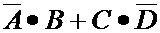is read as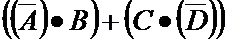.  The latter is really messy.

A·B + C·D = 1·0 + 1·1 = 0 + 1 = 1

A·(B + C)·D = 1·(0 + 1)·1 = 1 · 1 · 1 = 1=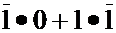= 0 · 0 + 1 · 0 = 0 + 0 = 0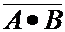=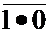=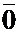= 1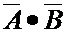=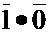= 0 · 1 = 0

The Basic Unusual Boolean Theorem

Here are two sets of theorems in Boolean algebra.

For all X      0·X     =      0              OK
For all X      1
·X     =      X             OK
For all X      0 + X   =      X             OK
For all X      1 + X   =      1              What?

Consider the following truth tables

 W X W + X 0 0 0 0 1 1 1 0 1 1 1 1

From this, we derive the truth table proving the last two theorems.

 X 0 + X 1 + X 0 0 1 1 1 1

The Principle of Duality

If a statement in Boolean algebra is true, so is its dual.

To take the dual of an expression do the following:
change all logical AND to logical OR and all logical OR to logical AND
change all 0 to 1 and 1 to 0.

Postulate                        Dual
0·X     =      0                  1 + X   =        1
1
·X     =      X                 0 + X   =        X
0 + X   =      X                 1
·X     =        X
1 + X   =      1                  0
·X     =        0

An Unexpected Pair:    Two distributive laws, each the dual of the other.

For all Boolean values of Boolean variables A, B, C:   A·(B + C) = A·B + A·C

For all Boolean values of Boolean variables A, B, C:   A + B·C = (A + B)·(A + C)

If A = 1, the statement becomes 1 + B·C = (1 + B)·(1 + C), or 1 = 1·1.

If A = 0, the statement becomes 0 + B·C = (0 + B)·(0 + C), or B·C = B·C.